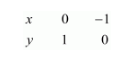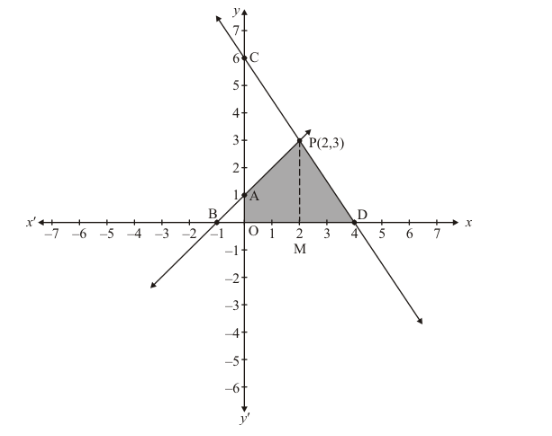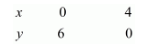# Draw the graphs of x − y + 1 = 0 and 3x + 2y − 12 = 0.`
Question:

Draw the graphs of x − y + 1 = 0 and 3x + 2y − 12 = 0. Determine the coordinates of the vertices of the triangle formed by these lines and x-axis and shade the triangular area. Calculate the area bounded by these lines and x-axis.

Solution:

The given equations are

$x-y+1=0$...$.(i )$

$3 x+2 y-12=0$$...(i i)$

Putting $x=0$ in equation $(i)$, we get:

$\Rightarrow 0-y=-1$

$\Rightarrow y=1$

$x=0, \quad y=1$

Putting $y=0$ in equation (i) we get:

$\Rightarrow x-0=-1$

$\Rightarrow x=-1$

$x=-1, \quad y=0$

Use the following table to draw the graph.Draw the graph by plotting the two points $A(0,1), B(-1,0)$ from table.$3 x+2 y=12$....(ii)

Putting $x=0$ in equation (ii) we get:

$\Rightarrow 3 \times 0+2 y=12$

$\Rightarrow y=6$

$x=0, \quad y=6$

Putting $y=0$ in equation $(i i)$. We get:

$\Rightarrow 3 x+2 \times 0=12$

$\Rightarrow x=4$

$x=4, \quad y=0$

Use the following table to draw the graph.Draw the graph by plotting the two points $C(0,6), D(4,0)$ from table.

The two lines intersect at $P(2,3)$.

Now, Required area = Area of shaded region

$\Rightarrow$ Required area $=$ Area of PBD

$\Rightarrow$ Required area $=1 / 2$ (basexheight)

$\Rightarrow$ Required area $=1 / 2(B D \times P M)$

$\Rightarrow$ Required area $=1 / 2(5 \times 3)$ sq. units

Hence the area $=7.5$ sq.units